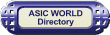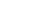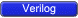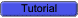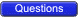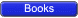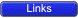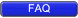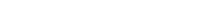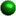Gate and Switch delays

In real circuits, logic gates have delays associated with them. Verilog provides the mechanism to associate delays with gates.• Rise, Fall and Turn-off delays.
• Minimal, Typical, and Maximum delays.In Verilog delays can be introduced with #'num' as in the examples below, where # is a special character to introduce delay, and 'num' is the number of ticks simulator should delay current statement execution.• #1 a = b : Delay by 1, i.e. execute after 1 tick
• #2 not (a,b) : Delay by 2 all assignments made to a.Real transistors have resolution delays between the input and output. This is modeled in Verilog by specifying one or more delays for the rise, fall, turn-on and turn off time seperated by commas.Syntax: keyword #(delay{s}) unique_name (node specifications);Switch element Number Of Delays Specified delays Switch 1 Rise, fall and turn-off times of equal length 2 Rise and fall times 3 Rise, fall and turn off (r)tranif0, (r)tranif1 1 both turn on and turn off 2 turn on, turn off (r)tran 0 None allowedRise Delay

The rise delay is associated with a gate output transition to 1 from another value (0, x, z).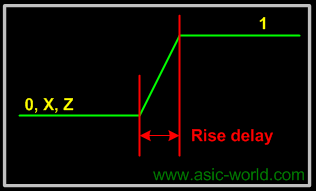Fall Delay

The fall delay is associated with a gate output transition to 0 from another value (1, x, z).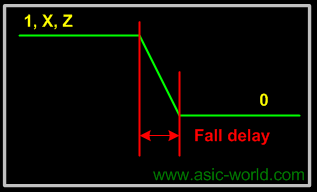Turn-off Delay

The Turn-off delay is associated with a gate output transition to z from another value (0, 1, x).Min Value

The min value is the minimum delay value that the gate is expected to have.Typ Value

The typ value is the typical delay value that the gate is expected to have.Max Value

The max value is the maximum delay value that the gate is expected to have.Example

Below are some examples to show the usage of delays.Example - Single Delay```
1 module  buf_gate ();
2 reg in;
3 wire out;
4
5 buf #(5) (out,in);
6
7 initial begin
8   \$monitor ("Time = %g in = %b out=%b", \$time, in, out);
9   in = 0;
10    #10  in = 1;
11    #10  in = 0;
12    #10  \$finish;
13 end
14
15 endmodule
`````` Time = 0 in = 0 out=x
Time = 5 in = 0 out=0
Time = 10 in = 1 out=0
Time = 15 in = 1 out=1
Time = 20 in = 0 out=1
Time = 25 in = 0 out=0
```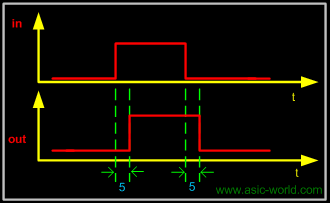Example - Two Delays```
1 module  buf_gate1 ();
2 reg in;
3 wire out;
4
5 buf #(2,3) (out,in);
6
7 initial begin
8   \$monitor ("Time = %g in = %b out=%b", \$time, in, out);
9   in = 0;
10    #10  in = 1;
11    #10  in = 0;
12    #10  \$finish;
13 end
14
15 endmodule
`````` Time = 0 in = 0 out=x
Time = 3 in = 0 out=0
Time = 10 in = 1 out=0
Time = 12 in = 1 out=1
Time = 20 in = 0 out=1
Time = 23 in = 0 out=0
```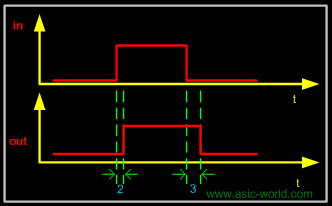Example - All Delays```
1 module delay();
2  reg in;
3  wire rise_delay, fall_delay, all_delay;
4
5  initial begin
6   \$monitor (
7     "Time=%g in=%b rise_delay=%b fall_delay=%b all_delay=%b",
8     \$time, in, rise_delay, fall_delay, all_delay);
9   in = 0;
10    #10  in = 1;
11    #10  in = 0;
12    #20  \$finish;
13  end
14
15  buf #(1,0)U_rise (rise_delay,in);
16  buf #(0,1)U_fall (fall_delay,in);
17  buf  #1  U_all (all_delay,in);
18
19 endmodule
`````` Time = 0 in = 0 rise_delay = 0 fall_delay = x all_delay = x
Time = 1 in = 0 rise_delay = 0 fall_delay = 0 all_delay = 0
Time = 10 in = 1 rise_delay = 0 fall_delay = 1 all_delay = 0
Time = 11 in = 1 rise_delay = 1 fall_delay = 1 all_delay = 1
Time = 20 in = 0 rise_delay = 0 fall_delay = 1 all_delay = 1
Time = 21 in = 0 rise_delay = 0 fall_delay = 0 all_delay = 0
```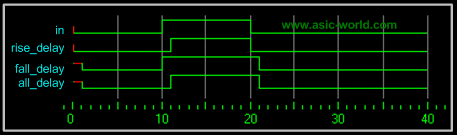Example - Complex Example```
1 module delay_example();
2
3 wire out1,out2,out3,out4,out5,out6;
4 reg b,c;
5
6 // Delay for all transitions
7 or      #5                    u_or     (out1,b,c);
8 // Rise and fall delay
9 and    #(1,2)               u_and    (out2,b,c);
10 // Rise, fall and turn off delay
11 nor    #(1,2,3)             u_nor    (out3,b,c);
12 //One Delay, min, typ and max
13 nand   #(1:2:3)             u_nand   (out4,b,c);
14 //Two delays, min,typ and max
15 buf    #(1:4:8,4:5:6)       u_buf    (out5,b);
16 //Three delays, min, typ, and max
17 notif1 #(1:2:3,4:5:6,7:8:9) u_notif1 (out6,b,c);
18
19 //Testbench code
20 initial begin
21   \$monitor (
22   "Time=%g b=%b c=%b  out1=%b out2=%b out3=%b out4=%b out5=%b out6=%b",
23     \$time, b, c , out1, out2, out3, out4, out5, out6);
24   b = 0;
25   c = 0;
26    #10  b = 1;
27    #10  c = 1;
28    #10  b = 0;
29    #10  \$finish;
30 end
31
32 endmodule
`````` Time = 0 b = 0 c=0  out1=x out2=x out3=x out4=x out5=x out6=x
Time = 1 b = 0 c=0  out1=x out2=x out3=1 out4=x out5=x out6=x
Time = 2 b = 0 c=0  out1=x out2=0 out3=1 out4=1 out5=x out6=z
Time = 5 b = 0 c=0  out1=0 out2=0 out3=1 out4=1 out5=0 out6=z
Time = 8 b = 0 c=0  out1=0 out2=0 out3=1 out4=1 out5=0 out6=z
Time = 10 b = 1 c=0  out1=0 out2=0 out3=1 out4=1 out5=0 out6=z
Time = 12 b = 1 c=0  out1=0 out2=0 out3=0 out4=1 out5=0 out6=z
Time = 14 b = 1 c=0  out1=0 out2=0 out3=0 out4=1 out5=1 out6=z
Time = 15 b = 1 c=0  out1=1 out2=0 out3=0 out4=1 out5=1 out6=z
Time = 20 b = 1 c=1  out1=1 out2=0 out3=0 out4=1 out5=1 out6=z
Time = 21 b = 1 c=1  out1=1 out2=1 out3=0 out4=1 out5=1 out6=z
Time = 22 b = 1 c=1  out1=1 out2=1 out3=0 out4=0 out5=1 out6=z
Time = 25 b = 1 c=1  out1=1 out2=1 out3=0 out4=0 out5=1 out6=0
Time = 30 b = 0 c=1  out1=1 out2=1 out3=0 out4=0 out5=1 out6=0
Time = 32 b = 0 c=1  out1=1 out2=0 out3=0 out4=1 out5=1 out6=1
Time = 35 b = 0 c=1  out1=1 out2=0 out3=0 out4=1 out5=0 out6=1
```N-Input Primitives

The and, nand, or, nor, xor, and xnor primitives have one output and any number of inputs• The single output is the first terminal.
• All other terminals are inputs.Examples```
1 module n_in_primitive();
2
3 wire out1,out2,out3;
4 reg in1,in2,in3,in4;
5
6 // Two input AND gate
7 and u_and1 (out1, in1, in2);
8 // four input AND gate
9 and u_and2 (out2, in1, in2, in3, in4);
10 // three input XNOR gate
11 xnor u_xnor1 (out3, in1, in2, in3);
12
13 //Testbench Code
14 initial begin
15   \$monitor (
16   "in1 = %b in2 = %b in3 = %b in4 = %b out1 = %b out2 = %b out3 = %b",
17   in1, in2, in3, in4, out1, out2, out3);
18   in1 = 0;
19   in2 = 0;
20   in3 = 0;
21   in4 = 0;
22    #1  in1 = 1;
23    #1  in2 = 1;
24    #1  in3 = 1;
25    #1  in4 = 1;
26    #1  \$finish;
27 end
28
29 endmodule
`````` in1 = 0 in2 = 0 in3 = 0 in4 = 0 out1 = 0 out2 = 0 out3 = 1
in1 = 1 in2 = 0 in3 = 0 in4 = 0 out1 = 0 out2 = 0 out3 = 0
in1 = 1 in2 = 1 in3 = 0 in4 = 0 out1 = 1 out2 = 0 out3 = 1
in1 = 1 in2 = 1 in3 = 1 in4 = 0 out1 = 1 out2 = 0 out3 = 0
in1 = 1 in2 = 1 in3 = 1 in4 = 1 out1 = 1 out2 = 1 out3 = 0
```N-Output Primitives

The buf and not primitives have any number of outputs and one input• The outputs are the first terminals listed.
• The last terminal is the single input.Examples```
1 module n_out_primitive();
2
3 wire out,out_0,out_1,out_2,out_3,out_a,out_b,out_c;
4 wire in;
5
6 // one output Buffer gate
7 buf u_buf0 (out,in);
8 // four output Buffer gate
9 buf u_buf1 (out_0, out_1, out_2, out_3, in);
10 // three output Invertor gate
11 not u_not0 (out_a, out_b, out_c, in);
12
13 endmodule
```